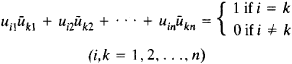# Unitary Matrix

Also found in: Dictionary, Wikipedia.
Related to Unitary Matrix: Normal matrix

## unitary matrix

[′yü·nə‚ter·ē ′mā·triks]
(mathematics)
A matrix whose inverse is equal to the complex conjugate of its transpose.

## Unitary Matrix

A unitary matrix of order n is an n × n matrix [uik] with complex entries such that the product of [uik] and its conjugate transpose [ūki] is the identity matrix E. The elements of a unitary matrix satisfy the relationsThe unitary matrices of order n form a group under multiplication. A unitary matrix with real entries is an orthogonal matrix.

References in periodicals archive ?
The unitary matrix [F.sub.1] is determined by the singular value decomposition (SVD) of [[??].sub.0] = U[summation][W.sup.T] and set [F.sub.1] = U.
where [summation] is a diagonal positive-definite matrix and Vis usually a unitary matrix.
Other examples result from choosing a unitary matrix Q and considering the system Q*HQx = Q*[e.sub.1].
A framework of the 4 x 2 unitary matrix [[PHI].sub.b] on [G.sub.4,2] was built similar to (4), and the degree of freedom of its elements was M(T - M) = 4.
The n x n unitary matrix [V.sup.T] denotes the conjugate transpose of the n x n unitary matrix V.
They cover unitary matrix ensembles, the Riemann-Hilbert problem for orthogonal polynomials, discrete orthogonal polynomials on an infinite lattice, introducing the six-vertex model, the Izergin-Korepin formula, the disordered phase, the anti-ferroelectric phase, the ferroelectric phase, and between the phases.
Thus, if [W] is a whitening matrix, [A'] = [W][A] is an [N.sub.m] x [N.sub.m] unitary matrix. As a consequence, the mixing matrix can be factored as the product of the inverse of the whitening matrix and a unitary matrix (to be determined).
In addition, we propose the channel gain matrix which has its singular value matrix in its middle as well as its eigen matrix and unitary matrix in its right or left side appropriately, which results in the new diagonal matrix.
Practical interest in the polar decomposition stems mainly from the fact that the unitary polar factor of A is the nearest unitary matrix to A in any unitarily invariant norm .
In , we developed a unitary matrix pencil (UMP) based delay estimation algorithm for 3D localization in UWB wireless sensor networks, which can reduce the complexity load.
Input: A, B and a dimension l Output: V which solves the trace ratio optimization problem (27) (1) Select an initial n x I unitary matrix V; (2) Compute [rho] = tr([V.sup.T]AV)/tr[[V.sup.T]BV]; (3) Until convergence Do: (3.1) Compute the I eigenvectors [{[v.sub.i]}.sup.t.sub.i=1] of the matrix A - [rho]B corresponding to the largest l eigenvalues.

Site: Follow: Share:
Open / Close